1.1 工作环境

1.1.1 R的历史与发展

1.1.2 R的资源

1.1.3 RGui

1.1.4 Rstudio

1.1.5 为什么选择R

1.1.6 Tips for R

1.1.6 Tips for R

1.2 工作环境

1.2.1 对象

1.2.2 数据类型

1.2.3 [向量]

1.2.4 [矩阵]和数组

1.2.5 [列表]

1.2.6 [数据框]

1.2.7 因子与表

1.2.8 表达式与公式

1.3 [对象的运算]

1.3.1 对象类型转换

1.3.2 运算符

1.3.3 运算函数

1.4 常用命令

1.4.1 工作目录与R内存

1.4.2 加载与保存

1.4.3 显示

1.4.4 挂接

# 1.1 工作环境

## 1.1.6 Tips for R

Rgui 设置 / 保存：将 Rconsole 文件保存在安装目录 etc 文件夹下

setwd("E:/大二下2020春/3R语言/attached for 1 and 2")
rm(list=ls()) #清除当前空间中所有对象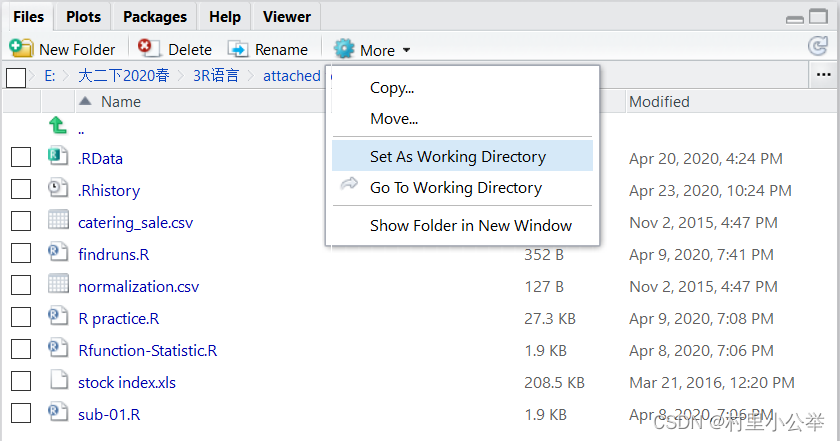?lm 查询lm函数的用法 ----- 函数用法 + 参数 + 例子

Q ：搜索查看 R 包：
Economertrics 计量经济学 Empirical Finance 实证金融 Time Series Analysis 时间序列分析
Q ：过去成功的代码无法运行或得到不同结果：

Q ：不同程序包市场有包含同名的函数：
eg. ipred adabag 包都有函数 bagging() ，因此要调用时不能同时打开这两个包
Q Rstudio 关联不上主程序：

Rstudio R 装同一目录下

Q ：只运行代码、不显示结果

# 1.2 工作环境

## 1.2.1 对象

1. 对象是 “R 创建与操作的实体
2. 工作空间：当前路径下存储的所有对象的组合

rm(list=ls()) #清除当前空间中所有对象
3. 对象命名：数字不开头，大小写敏感，不同关键字

## 1.2.2 数据类型

1. 对象有两个属性：类型 class() ，长度 length()
2. NA 缺失值 NULL 空值
3. 5 种基本数据类型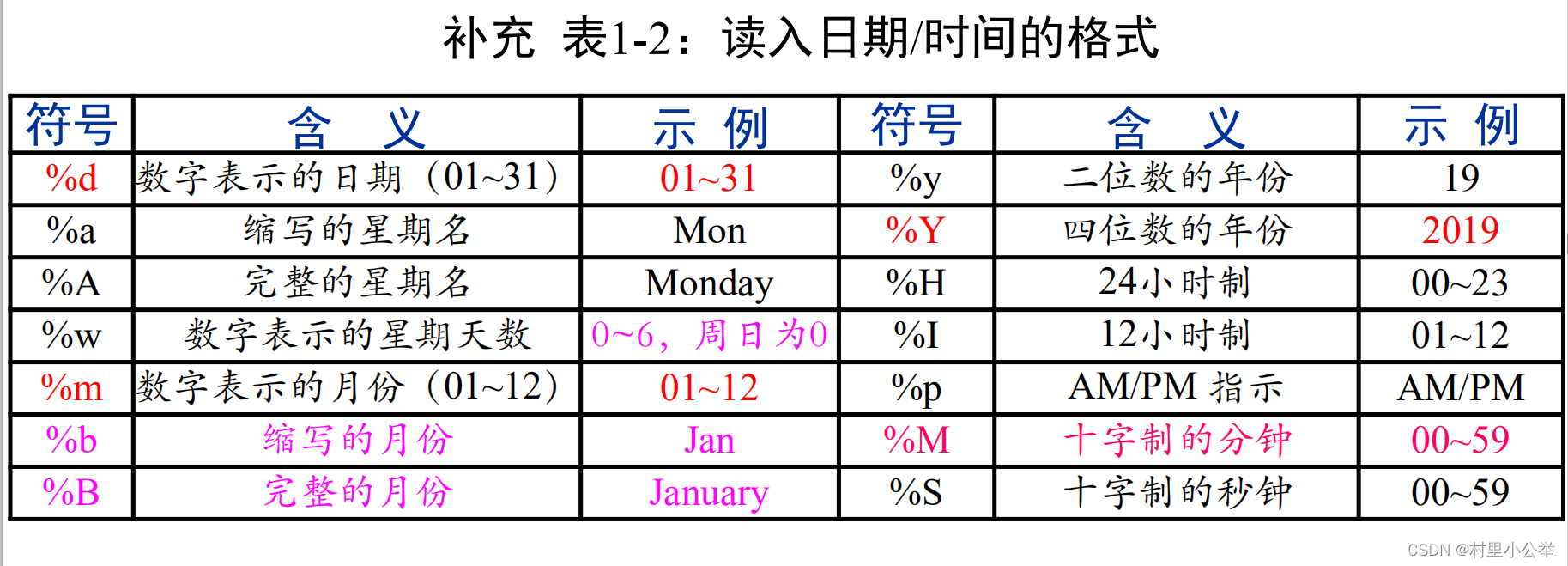#(1)numeric
x<-12;length(x);class(x);
#(2)character
z<-"Display \"a\" string" #字符需要用单双引号引起来
w<-'Display "b" string' #双引号中的双引号需要用反斜杠'\'区分开
cat(z,w) #concatenate and print 连接并打印
#(3)logical
y<- TRUE;class(y);length(y);
#(4)complex
m<-2+3i;class(m);length(m); #长度为1
#(5)时间型，日期/时间变量(***)
(dates <- c("01/27/2016", "02/27/2016", "01/14/2016", "02/28/2016",
"02/01/2016")) #最外层括号显示运行结果
is.numeric.Date(dates) #是否是数值日期变量
is.character(dates) #是否是字符日期变量
(date <- as.Date(dates, format = "%m/%d/%Y"))
#按照（format月日年）的格式*读入*日期，进行年月日的日期变量转换(输出：年-月-日)
#as.Date()只能转换“年月日星期”的字符日期，不能转换具体到“时分秒”的字符时刻
#日期变量，可以直接做运算
(dateform <- format(date, "%m/%d/%Y")) # 日期变量，转换为“任意指定格式”的*字符串*
class(date)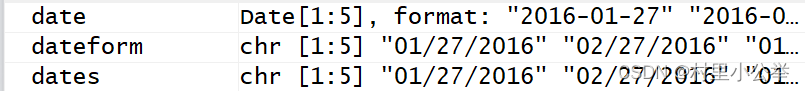#将“字符时间”，转换为“可计算的时间变量”(**)
x <- c("2016-02-08 10:07:52", "2016-08-07 19:33:02")
is.character(x) # 判定是否为字符型变量
as.POSIXlt(x, tz = "", format = "%Y-%m-%d %H:%M:%S")
#POSIXct格式的时间：以有符号整数形式存储，表示从1970-01-01到该时间点经过的秒数
#POSIXlt格式的时间：以字符串形式存储，包含年月日等
# "05/27/84" 对应格式 "%m/%d/%y"
# "May 27 1984" 对应格式 "%B %d %Y"
#CST,为中国标准时区tz
(x <- strptime(x, "%Y-%m-%d %H:%M:%S", tz = ""))
# 按“年月日 时分秒”转换为时间变量，与as.POSIXlt()等效、但格式略有不同
#将“时间变量”转换为“字符日期”(***)
strftime(x, format = "%m/%d/%Y") #按format设定个事逆转为字符串日期，不保留具体的时分秒
format(x, "%Y-%m-%d %a %b") #输出格式，转换成format指定的字符串日期
#缩写星期，简化月份

format() 将其他类型变量转换成字符串
date < - as.Date(dates, format = "%m/%d/%Y") 年月日转换成日期变量
as.POSIXlt(x, tz = "", format = "%Y - %m - %d %H:%M:%S")
(x < - strptime(x, "%Y - %m - %d %H:%M:%S", tz = ""))

## 1.2.3 [向量]

1. 一维数组，核心
2. R/S 中无标量（标量即 x ），通过各种 class 的向量来存储数据，矩阵数组因子实质上是向量
3. 连接函数 c()
4. 生成规律序列

：的优先级高于 * 小于 ^

seq(1, -9) # 只给出首项和尾项数据，by自动匹配为1或-1
seq(1, -9, length.out = 5) # 给出首项、尾项、长度，自动计算等差
seq(1, -9, by = -2) # 给出首项、尾项、步长，自动计算长度
seq(1, by = 2, length.out = 10) # 给出首项、步长、长度，自动计算尾项
#by步长，不引起混淆是length.out可以记为length


# Replicate：“重复生成”某一元素或对象
rep(1:3, 2) #times重复两次
rep(1:3, each = 2) #each每个重复两次
rep(1:3, c(2, 1, 2)) #按照规定指定重复每个元素
rep(1:3, each = 2, length.out = 4) #限制输出个数
rep(1:3, each = 2, times = 3) #序列中“各个元素”分别重复两次，“整个序列”重复3次
rep(as.factor(c("因子1", "因子2", "因子3")), 3) #因子定义见后文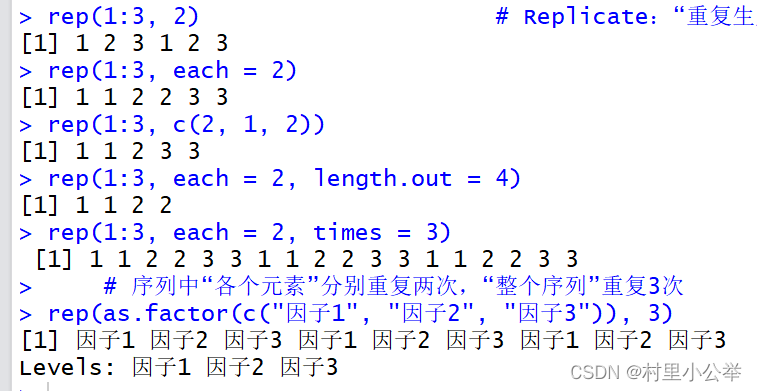5. 基于向量的 R 编程的核心 **

#“中括号 + 下标”索引(元素的值)
vector <- c(11, 12, 13, 14)
vector[c(1, 4)] #11 14
vector[-c(1:3)] #14
vector[c(TRUE, TRUE, FALSE, FALSE)] #11 12
#名称索引
names(vector) <- c("one", "two", "three", "four") #命名字
vector[c("one", "two", "four")] #11 12 14


subset() : [] 类似，区别在于会自动剔除 NA 值（筛选出的结果相同）
#subset索引
vector <- c(11, 12, 13, 14)
subset(vector, vector > 11 & vector < 14) # 检索向量中满足条件的元素

which ：查找位置
#which索引“满足逻辑条件的元素位置”(***)
vector <- c(11, 12, 13, 14)
which(vector == 13)
which(vector == c(11, 14)) # 向量中等于11和14的元素所在的位置
which(vector != 12)
which(vector > 12 & vector < 14)
which.max(vector)
which.min(vector)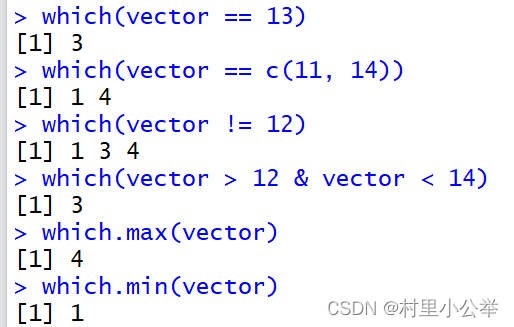match() 返回向量 x 是否是 table 中的任一个值，有则返回在 table 中的位置，不匹配的返回
NA
## match方式索引
vector <- c(11, 12, 13, 14)
match(vector, c(14, 11, 13)) # 2 NA 3 1
match(vector, c(11, 14)) # 1 NA NA 2
vector[match(vector, c(11, 14))] # 11 NA NA 12

%in% 值匹配
## 值匹配符
vector <- c(11, 12, 13, 14)
c(11, 18) %in% vector #TRUE FALSE 11在vector中,18不在


6. 变量 x 不需要声明，只是一个指针，不同时间指向不同对象

8. tips

R 生成等比数列：将等差数列放在指数项上
9. 向量的增删
##增
x<-x <- c(1, 2, 3, 4)
(x <- c(x, c(7, 8, 9)))
(x <- append(x, 5:6, after=4)) #1 2 3 4 5 6 7 8 9 在4后添加5和6
##删
x <- c(1, 2, 3, 4,5,6,7)
(x <- x[-c(3:5)]) #删除3,4,5号元素 
10. 向量的排序
##sort
x <- c(5, 6, 8, 7, 4, 1, 9)
x1 <- c("B", "A", "C")
x2 <- c(3, 2, NA, 1, 4, 5)
sort(x) # 1 4 5 6 7 8 9
sort(x, decreasing = TRUE) # 9 8 7 6 5 4 1
sort(x1) # "A" "B" "C"
sort(x2, na.last = TRUE) # 1 2 3 4 5 NA
##order返回元素
x <- c(5, 6, 8, 7, 4, 1, 9)
order(x) #4原来在5的位置 6 5 1 2 4 3 7
x[order(x)] #1 4 5 6 7 8 9
rev(x) 倒序（按位置）

## 1.2.4 [矩阵]和数组

1. 带有多个下标、同一类型元素的集合、向量筛选子集、与向量化运算
2. 生成行列 martix() 行表示观测值，列表示变量

## 创建矩阵
x <- c(1:10) # 创建一个向量，作为矩阵的数据
(a <- matrix(x, nrow = 5, ncol = 2, byrow = T))
# 创建一个矩阵，定义矩阵的行数为5、列数为2，按行读取数据
(b <- matrix(x)) # 10行1列
dim(b) <- c(5, 2) # 5行2列
b
(c <- matrix(x, nrow = 5, ncol = 2, byrow = F,
dimnames = list(c("r1", "r2", "r3", "r4", "r5"), c("c1","c2"))))
length(c)

length 返回矩阵元素的个数
3. 索引 M[ 行下标 ( 或范围 ) ，列下表 ( 或范围 )]

##矩阵索引/筛选：“中括号 + 下标范围”
x <- c(1:10)
(a <- matrix(x, nrow = 5, ncol = 2, byrow = F,
dimnames = list(c("r1", "r2", "r3", "r4", "r5"), c("c1","c2"))))
a[1, ] # 检索第一行
a[, 1] # 检索第一列
a[c(3:5), ] # 检索第3-5行
a[-c(2, 4), 2] # 默认drop值为真，将返回一个“维数尽可能低的对象”(此处为向量)
#r1 r3 r5
# 6 8 10
a[-c(2, 4), 2, drop = F] #去掉第2,4行，提取第二列，但索引是单因子矩阵，不允许降维
# c2
#r1 6
#r3 8
#r5 10
a[a[, 1]>3, ] # 筛选条件为“比较表达式”时，依逻辑布尔值为真、筛选出“需要的矩阵的行或


4. 筛选：比较表达式，结果为布尔值向量
5. 编辑

rbind() 横向合并 cbind() 纵向合并

##矩阵转换为向量
x <- c(1:10)
(a <- matrix(x, ncol = 2, nrow = 5, byrow = T))
(b <- as.vector(a)) # 将矩阵“转化为向量”
# 1 3 5 7 9 2 4 6 8 10
matrix(b, nrow=2)
# [,1] [,2] [,3] [,4] [,5]
#[1,] 1 5 9 4 8
#[2,] 3 7 2 6 10
##矩阵转换为向量
(a <- matrix(1:10, nrow = 5, ncol = 2))
(a1 <- rbind(a, c(11,12))) # 按行的形式合并
(a2 <- cbind(a, c(11:15)))
(a3 <- rbind(a, 1)) #短向量元素不足，按循环补齐后绑定
(a4 <- cbind(a, 1))
(a5 <- a[-1, ]) # 删除第一行
(a6 <- a[, -2]) # 删除第二列 
6. 三个常见的矩阵运算
##求和和均值
colSums_A <- colSums(A)
colMeans_A <- colMeans(A)
rowSums(A)
rowMeans(A)
#没有rowvar()函数，可以用apply()函数解决


(A <- matrix(c(1:9), ncol = 3, nrow = 3))
diag_A <- diag(A) # 矩阵取对角(1,5,9)
diag(diag_A)
# [,1] [,2] [,3]
#[1,] 1 0 0
#[2,] 0 5 0
#[3,] 0 0 9


##生成三角矩阵
(Tri <- matrix(1:25,5,5)) # 简化形式、指定行/列
(upper.tri(Tri))
Tri[upper.tri(Tri)] <- 0
Tri 
7. 生成数组

x <- c(1:30)
dim1 <- c("A1", "A2", "A3")
dim2 <- c("B1", "B2", "B3", "B4", "B5")
dim3 <- c("C1", "c2")
(a <- array(x, dim = c(3, 5, 2), dimnames = list(dim1, dim2, dim3)))


## 1.2.5 [列表]

1. 列表的创建

g <- "My List" ; class(g)
h <- c(25, 26, 18, 39) ; class(h)
j <- matrix(1:10, nrow = 5) ; class(j)
(mylist <- list(title = g, ages = h, j))
summary(mylist)
names(mylist)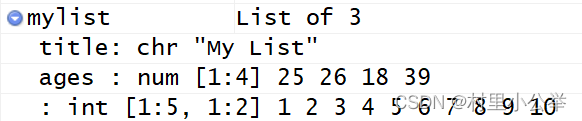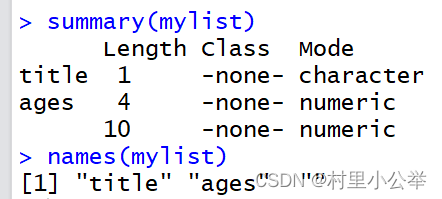unlist<-unlist(mylist)
# title ages1 ages2 ages3 ages4
#"My List" "25" "26" "18" "39" "1" "2"
#
# "3" "4" "5" "6" "7" "8" "9"
#
# "10"
mode(unlist) ; class(unlist) ; typeof(unlist)
# "character" 一般情况下全部转换为字符向量


2. 索引和元素提取
5 种类）
tips: 下标 / 元素名 + / 双括号 $元素名 Lt[[ 下标 ]] 取出列表元素 Lt[[ 下标 ]] 取出字列表 Lt[[" 元素名称 "]] Lt[" 列表元素 "] 通过 列表名$ 元素名 引用列表中的元素（效果与双重中括号相同）
(data <- list(a = c(1, 2, 3, 4), b = c("one", "two", "three"),
c = c(TRUE, FALSE), d = (1 + 2i)))
#Lt[[下标]]：双重中括号，取出的是“列表元素”（不再含元素/变量名） Lt[["a"]]同理
data[] ; class(data[])
#Lt[下标或下标范围]：单重中括号，取出的是“子列表” Lt["a"]同理
data ; class(data)
#去第一列第2,4元素值
data[][c(2, 4)]

2. R 函数的返回值大多都是列表（组织调用完全不相干的信息）
3. 列表的编辑

(data <- list(a = c(1, 2, 3, 4), b = c("one", "two", "three"),
c = c(TRUE, FALSE), d = (1 + 2i)))
##添加
#赋值语句
data$e<-5:8 #连接函数 (data1 <- c(data, list(e = c(5, 6, 7, 8)))) (data2 <- c(data, e = list(c(5, 6, 7, 8)))) ##更改（对已有操作同上即为更改） ##删除（赋值NULL） data$b <- NULL ; data


## 1.2.6 [数据框]

1. 创建数据框 data.frame( 组件 1,... 组件 n,stringAsFactor=F)

### 6.数据框(***)：创建、索引、编辑
## 1) 创建数据框：每列数据类型可不同，但长度必须相同
## 数据框，是一种特殊列表，以“矩阵形式”存放数据
#eg1
data_iris <- data.frame(Sepal.Length = c(5.1, 4.9, 4.7, 4.6),
Sepal.Width = c(3.5, 3.0, 3.2, 3.1),
Petal.Length = c(1.4, 1.4, 1.3, 1.5),
Petal.Width = rep(0.2, 4))
data_iris
#eg2
(data_matrix <- matrix(1:8, c(4, 2))) # 创建一个矩阵
(data.frame(data_matrix))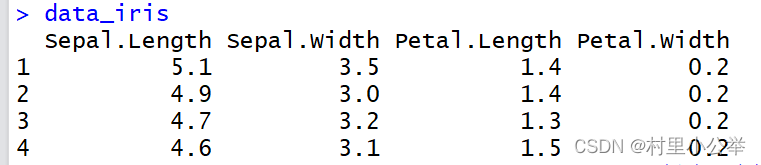2. 索引数据框

3 种双重括号的方法）

##列索引
data_iris[, 1] # 矩阵索引第一列（有逗号—>矩阵方式）
data_iris[] # 列表索引第一列
data_iris$Sepal.Length # 列表按元素名索引 data_iris["Sepal.Length"] # Sepal.Length #1 5.1 #2 4.9 #3 4.7 #4 4.6 class(data_iris["Sepal.Length"]) #"data.frame" ##行索引(用矩阵的方式) ##单个元素索引 data_iris$Sepal.Length # 先索引出列，取第一个
data_iris["Sepal.Length"] # 子数据框为一个元素
# Sepal.Length
#1 5.1
#2 4.9
#3 4.7
#4 4.6
## subset函数索引(**)
subset(data_iris, Sepal.Length < 5) #只有第一行大于5
data_iris[data_iris$Sepal.Length < 5, ] #类似矩阵处理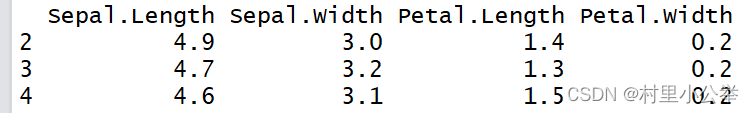3. 数据框的其他矩阵式操作 数据框的筛选 df[ 比较表达式 ] 可以多个表达式布尔结果的运算 缺失值处理，例如： mean(na,rm=T) 或直接调用 na.omit(df) 或调用 complete.cases(df) 检查每行数据是否完备 合并 rbind() cbind() 合并 / 增添向量 (data_iris <- rbind(data_iris, list(5.0, 3.6, 1.4, 0.2))) (data_iris <- cbind(data_iris, Species = rep("setosa", 5)))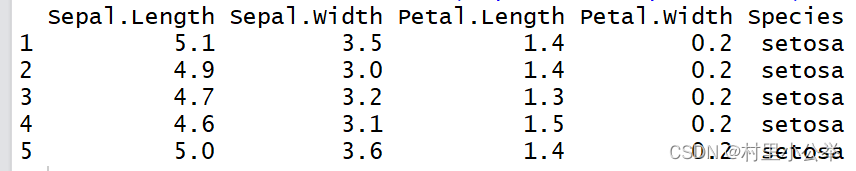apply(df,1,f) 对第 1 行进行 f 运算 数据框的删除同矩阵 [ - 索引值 ] 数据框的行列名字 ##查询 names(data_iris) # 查看数据框的“列名” ；注意，不是“行名” ##修改 names(data_iris) <- "sepal.length" #修改列名 rownames(data_iris) <- c("one","two","three","four", "five") #修改行名  4. 数据框合并 关系数据库：依照某个共同变量的相同值，组合成一张表 merge(df1,df2,by.x=tag1,by.y=tag2) tag1 tag2 ：匹配变量，含有相同信息，但组件名和 标签不同 merge(df1,df2,by=c(KeyField1,KeyField2)) 多个共同变量匹配 ## 1.2.7 因子与表 1. 因子和水平 创建 factor() 用于分类，计数； ordered 参数可以控制因子是否有序 因子向量中不同值的记录成为水平 levels() 因子的长度是指数据的长度，而不是水平的个数 因子水平数据的频数分布表 table() ，按因子个数分为一维频数表、二维列联表 创建因子变量 gl() 使用于因子水平反复情况（不常用） 2. 因子、表相关的操作函数 tapply(x,f,g) 先依因子 f ，对向 x 进行 分组 f,x 等长），每组对应一个因子水平，再对分 组后的 x 的子向量， 应用函数 g() f 还可以是多个引子的列表 list(f1,f2...) ，对 x 中的数据进行交叉分组 split(x,f) 只依因子 f ，对数据对象 x 进行 分组 x: 向量、数据框 by(x,f,g) tapply 相比，既可以向量也可以用于数据框 aggregate(df,Lt(f),g) 对列表因子产生的、分组数据框中的每个变量都进行函数 g 运算 cut(x,breaks) 生成区间分类因子向量 ### 7. 因子(*)：类别 + 有序 ## 1) 创建因子 # 离散型变量，往往用于“分类、或计数”，需要借助因子对象来分析计算 # 因子，可简单视为“附加了额外信息的[向量]”，分为“类别 + 有序” factor(1:4) factor(1:4,levels=1:2) #数字1仅表示因子水平1 factor(c((1:4),(4:1)),labels=c("A","B","C","D"))# labels：因子水平的标签、用数字或字 母无本质区别 (ff <- factor(substring("statistics", 1:10, 1:10), levels = letters))# 将文本/字符串statistics“逐个拆分”为单个字符、创建因子向量，水平为26个小写字母 (f <- factor(ff)) ff[, drop = TRUE] # 等价于 f <- factor(ff) factor(letters[1:20], labels = "letter") # 创建因子向量，“水平名称/标签”为letter (z <- factor(LETTERS[7:1], ordered = TRUE))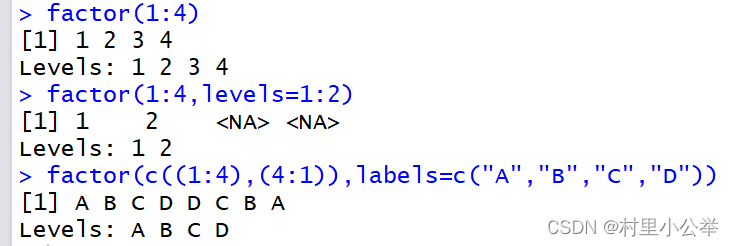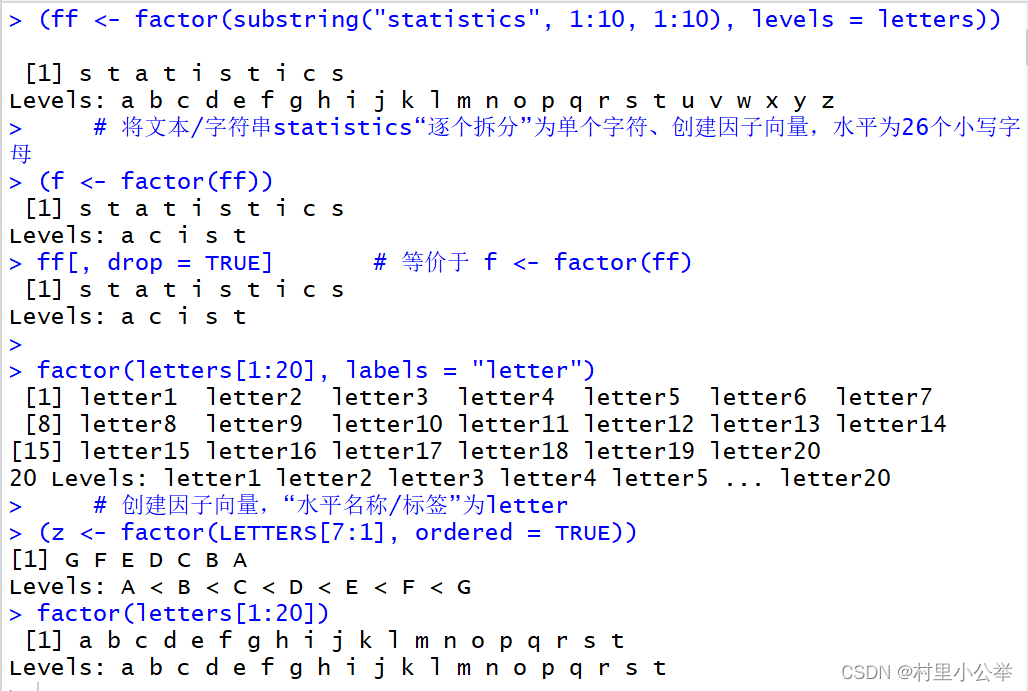gl(n, k, length) n为水平数，k为重复次数 ## 创建“水平/level反复重复”的因子：gl(n, k, length) gl(3, 3) # 1 1 1 2 2 2 3 3 3 #Levels: 1 2 3 gl(2, 3, labels = c("TRUE","FALSE")) # 生成水平为“TRUE”和“FALSE”，每个水平重复3次的因 子序列 # TRUE TRUE TRUE FALSE FALSE FALSE #Levels: TRUE FALSE gl(2, 1, 10) # 1 2 1 2 1 2 1 2 1 2 #Levels: 1 2 gl(2, 2, 10) # 1 1 2 2 1 1 2 2 1 1 #Levels: 1 2 gl(3, 3, ordered = TRUE) # 生成水平数为3，每个水平重复3次的有序因子序列 # 1 1 1 2 2 2 3 3 3 #Levels: 1 < 2 < 3 ## 2) 水平的频数统计，因子存储方式 status <- c("Poor", "Improved", "Excellent", "Excellent", "Poor", "Excellent") #字符串 (status.factor <- factor(status, ordered = TRUE)) # 创建有序因子序列，按英文首字母、排 序因子水平 levels(status.factor) # "Excellent" "Improved" "Poor" table(status.factor) #频数分布表 #status.factor #Excellent Improved Poor #按因子个数可以扩展为2维，3维 # 3 1 2 class(status.factor) # 查看“数据对象的类型” # "ordered" "factor" mode(status.factor) # 储存是整数 # "numeric" typeof(status.factor) # 因子是按“整数”*存储*的，仅仅是“计数”分类的类别 # "integer" as.numeric(status.factor) # 3 2 1 1 3 1  其与应用将在后续展开 ## 1.2.8 表达式与公式 1. 表达式 不求值的表达式 expression() ，求值表达式 eval(expression()) 求偏导 D(f,x1) 2. ~ 表示 公式 例如 y~model model 中的变量： + 表示连接， : 表示交互作用， * 表示 a+b+a:b - 表示去掉 多项式拟合时，二次以上的项需要放入 I() 模型估计指定数据框 df 后， y~ . 表示数据框中除 y 以外 set.seed(12345) # 设置随机数的种子数 p1 <- rnorm(100) p2 <- runif(100) model.lm <- lm(p2~p1+I(p1^2)+I(p1^3)) # 公式，用操作符“~”简示：y~x # 多项式拟合时：二次以上的项，放入“ I() ”中 summary(model.lm)  回归结果的具体内容后续展开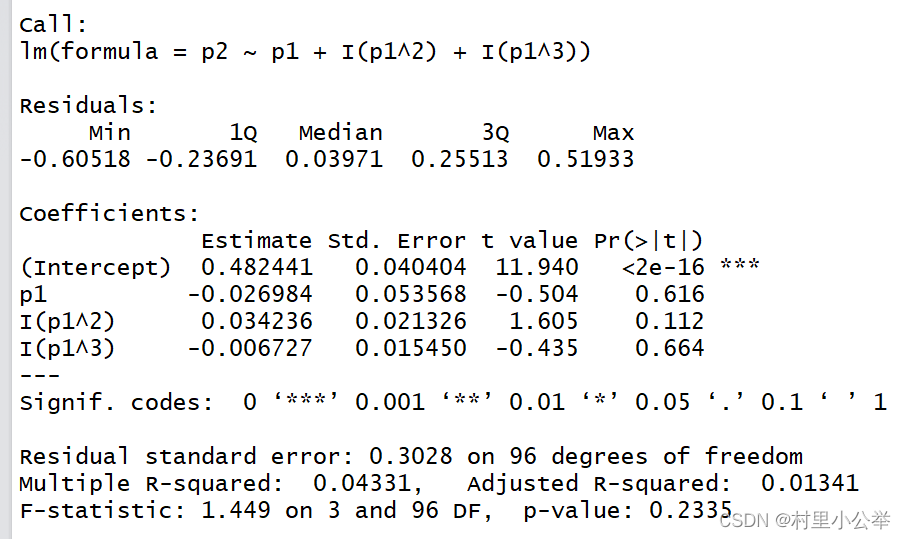# 1.3 [对象的运算] ## 1.3.1 对象类型转换 1. 对象类型的查看与判断 查看对象类型： class() 查看对象基本要素类型 mode() is. 数据类型 () 判断对象类型 is.numeric() is.matrix() (M <- matrix(1:12,nrow=3,ncol=4)) mode(M) # 模式：基本数据类型为“数值型” class(M) # 类：构造的对象类型为“矩阵” 数据对象结构的分类->面向对象 typeof(M) # 类型：细化数据类型为“整型” 类似mode，但更精细 is.matrix(M) # 判断数据对象类型 is.numeric(M) M.frame <- as.data.frame(M) # 默认：行是“观测点”，列为“元素/变量/字段/组件名”  2. 对象类型的转换 as. 数据类型 () 进行类型转换 数值型因子转化为数值型向量，若要保留最初设定的数字值，要先转换成字符串，再转换为 数值型，否则会变为 1 2 ... as.numeric(as.character(ff)) ff< - c(20200805,20200866) 连接函数 c() 遇到不同数据类型时，将会降维转换成同一类型，保持共性， list 被认为有较低 的优先级 methods(is) methods(as) 查询类型判断，类型转换函数 ## 1.3.2 运算符 1. 数学运算符：变量运算 + - */^ 取余： %% 整除 %/% 矩阵运算 * 和矩阵乘法 %*% kronecker kronecker(A,B) # y (m × n) 维， z (p × q) 维，则新矩阵维度： (mp × nq) #生成上三角矩阵 z <- matrix(c(11:19),ncol=3) z[lower.tri(z)] <- 0 2. 比较运算符：单个元素比较 < > <= >= == != 逐个比较 对象整体比较 identical() all.equal() （若不完全相等，后者可以返回平均 值相对差异） 3. 逻辑运算符：非 ! & && 表示只作用于对象的第一个元素） | || （类似 & 异或 xor(x,y) 4. 其他运算符： : 生成序列，优先级高于 * 小于 ^ 例： (x = pi*1:3^2) x %in% table 值匹配运算 多个回归变量用 + 连接、分隔 ## 1.3.3 运算函数 1. 向量运算函数 输入必须为向量，短向量重复利用，输出可以是数值或向量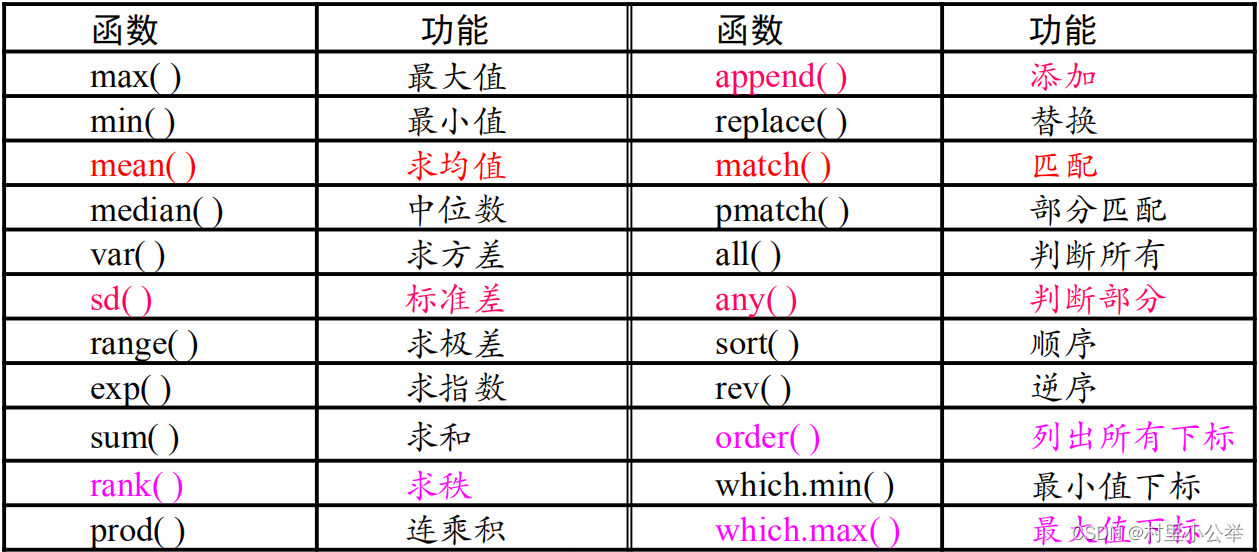x <- c(1,5,4,7,9) order(x) # 1 3 2 4 5 append(x, 2:3, after=2) # 在x的“指定下标位置”处、添加新的向量元素2:3 # 1 5 2 3 4 7 9  2. 矩阵运算函数 矩阵维度：行 / 列二维查看， dim() ；行数， nrow() ；列数， ncol() 矩阵合并：纵向按行， rbind() ；横向按列， cbind() svd() ，奇异值分解； qr() QR 分解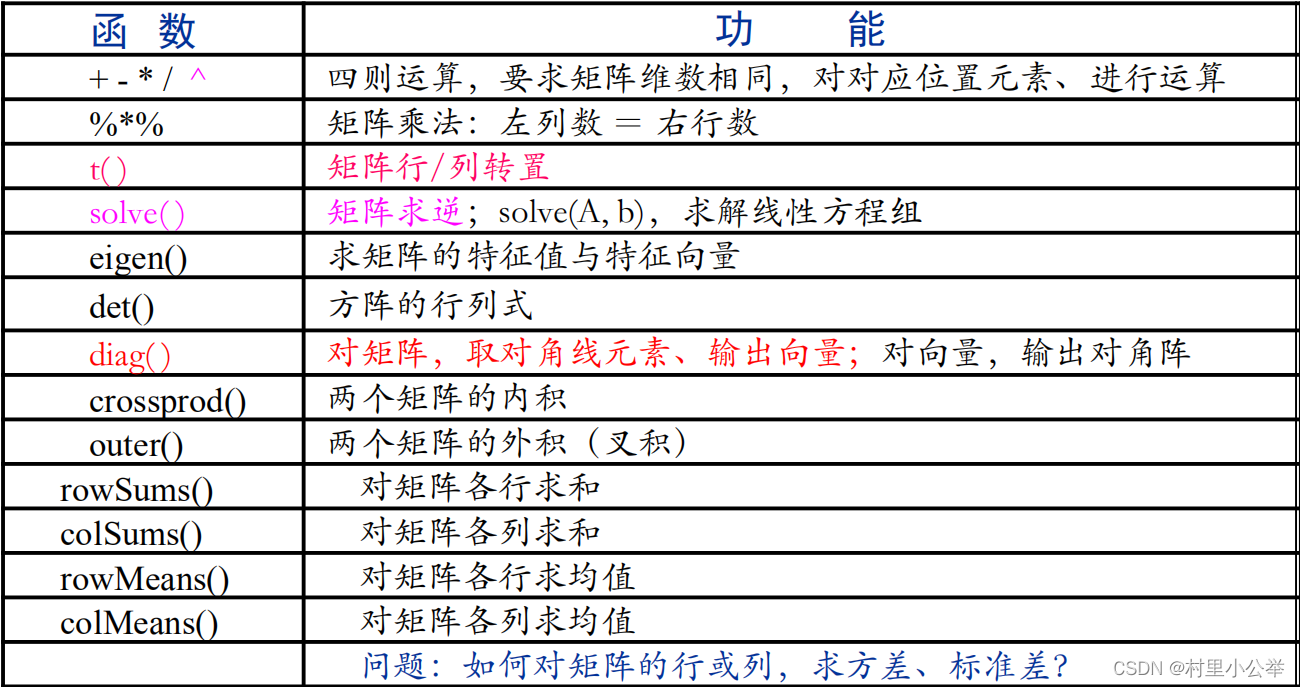(A <- matrix(sample(1:100, 16), ncol=4)) aperm(A,c(2,1)) # 数组"维度转换"函数，改行效果同转置 solve(A) # 矩阵求逆 ##矩阵合并 (x <- runif(10,-1,1)) (y <- rnorm(10,0.5,1)) (xy <- cbind(x,y)) # x y # [1,] 0.4480469 0.8775397 # [2,] 0.7246093 2.8934495 # [3,] -0.9006359 1.6335336 # [4,] -0.1471617 0.3429806 # [5,] 0.2373294 2.1354869 # [6,] -0.3626603 0.4452315 # [7,] -0.4686020 1.1615278 # [8,] 0.4733459 0.7969101 # [9,] -0.5738643 3.1170615 #[10,] -0.2144737 -0.7054672  3. apply 函数组：重复操作、批量运算 用于矩阵 apply(M,margin,fun) margin 表示固定某些维度不变，取 1 表示对矩阵 运算，取 2 表示对矩阵 运算 tapply(M,index,fun,...,simplify=TRUE) index 是因子列表对象。若是单因子向量，则数值长度与向量 x 等长 tapply(x,f,g) 若多音字运 算，则每个因子 fi 长度与向量长度一致 simplify 默认返回 table aggregrate() by(x,f,g) 突破 tapply() 函数对象为向量的限制，扩展为数据框 lapply() sapply() 对列表 / 数据框对象，对列表 Lt 的每个组件运算 f 函数 sweep() 批量运算函数 apply 函数主要用于 矩阵 ##apply函数 (x <- matrix(1:20, ncol = 4)) # [,1] [,2] [,3] [,4] #[1,] 1 6 11 16 #[2,] 2 7 12 17 #[3,] 3 8 13 18 #[4,] 4 9 14 19 #[5,] 5 10 15 20 apply(x, 1, mean) # 计算各行的均值 apply(x, 2, sd) # 计算各列标准差  tapply 函数主要用于 依照因子分类的向量 f1 <- factor(rep(1:4,length=14),levels=1:5,labels=c("A","B","C","D","E")) # 产生长度为14的因子对象，5个因子水平的标签、设为ABCDE f1 #  A B C D A B C D A B C D A B #Levels: A B C D E (x2 <- c(1:14)) tapply(x2,f1,sum) # f1是用于分类的“因子对象”，其长度与“向量x2”的[长度一致]，以便一一对应(*) # 先依照f1对x2进行分组，每组对应一个因子水平，对分组后x2的子向量，应用函数sum # 如A因子水平对应的子向量元素为1,5,9,13,它的和为28 # 默认返回简化结果table而不是list # A B C D E #28 32 21 24 NA aggregate(x2, by=list(f1),sum) #aggregate还可以对数据框查找 # Group.1 x #1 A 28 #2 B 32 #3 C 21 #4 D 24 by(x2, f1, sum) #f1: A # 28 #---------------------------------------------------- #f1: B # 32 #---------------------------------------------------- #f1: C # 21 #----------------------------------------------------#f1: D # 24 #---------------------------------------------------- #f1: E # NA tapply(x2,f1,sum,simplify=FALSE) #$A
# 28
#
#$B # 32 # #$C
# 21
#
#$D # 24 # #$E
#NULL

lapply 函数主要用于 列表 / 数据框
(L1 <- list(a=1:20, b=runif(30,-2,5), d=matrix(c(1:20),ncol=4)))
lapply(L1,quantile)
#对列表的“各个元素/字段/组件”，分别求分位数函数运算 (矩阵也是一种向量)(看做)
#$a # 0% 25% 50% 75% 100% # 1.00 5.75 10.50 15.25 20.00 # #$b
# 0% 25% 50% 75% 100%
#-1.90874464 -0.05957505 1.76850576 3.62932450 4.88275599
#
#$d # 0% 25% 50% 75% 100% # 1.00 5.75 10.50 15.25 20.00  sapply 函数主要用于 列表 / 数据框 ，是 lapply 的特殊形式 (L1 <- list(a=1:20, b=runif(30,-2,5), d=matrix(c(1:20),ncol=4))) sapply(L1,quantile,simplify=FALSE,use.names=FALSE) # 返回一个列表（此时同lapply） sapply(L1,quantile,simplify=TRUE,use.names=FALSE) #返回矩阵结果 # a b d #0% 1.00 -1.6077317 1.00 #25% 5.75 -0.1072232 5.75 #50% 10.50 1.4300179 10.50 #75% 15.25 2.5263637 15.25 #100% 20.00 4.6659900 20.00 (L2 <- list(c("a", "b", "c"), c("A", "B", "C"))) sapply(L2, paste, 1:3, sep="",simplify = TRUE) #sep为空表示没有a和1中无分隔符 # [,1] [,2] #[1,] "a1" "A1" #[2,] "b2" "B2" #[3,] "c3" "C3" sapply(L2, paste, 1:3, simplify = F) #sep默认为空格 #[] # "a 1" "b 2" "c 3" # #[] # "A 1" "B 2" "C 3"  # 1.4 常用命令 ## 1.4.1 工作目录与R内存 setwd() 设置当前工作目录 tips: / 或者 \ \ getwd() 查看当前工作目录 memory.size() 查看与增大工作内存 ## 1.4.2 加载与保存 library() 包加载 ; search() 查看当前空间中已经存在的包 ##下载包 install.packages("quantmod") ##加载包 library(xts) library(zoo) library(TTR) library(quantmod) # 加载quantmod的包 ##下载包 install.packages("car") ##加载包 library(carData) library(car) some(iris) #iris是一个自带的数据集  函数加载：包中的函数直接调用，自编函数需用 source(file=" 路径 / 函数名 .R") 加载 save() save.image() 保存工作空间对象，生成 RData 文件； jpeg() 保存图片 (x <- rnorm(10)) (y <- matrix(1:20, nrow=5)) (z <- paste("x", 1:10, sep='')) ls() # 查看当前空间中已存在的对象 save.image() # 保存工作空间所有对象，生成“ .RData”文件 save(x,y,file="S.RData") # 仅保存部分“指定的对象” load(file="S.RData")  ## 1.4.3 显示 head() 显示前六行 ； tail() 显示后六行 car 包中的 some() 任意行 print() ( 对象 ) 显示全部 关于四舍五入 E1 <-3.1415926535 E2 <-3.1415926535 (E1.r <- round(E1,digits=4)) # 四舍五入，小数点后保留四位 E1.r*1000000 # 3141600 options(digits=4) ; E2 # 显示四位数字，但未作四舍五入 E2*1000000 # 3141593  ## 1.4.4 挂接 attach() 调用数据框与列表中的元素 / 变量，将其挂接到内存中可以直接调用 detach() ; list() / unlist() ; #4.挂接命令 df <- data.frame(name=c("ZhangSan","XiaoHong","LiSi","XiaoLan"), sex=c("M","F","M","F"),age =c(20,21,19,20),weight=c(110,90,128,102)) attach(df) # 将“数据框/列表中的变量/元素”、直接“挂接到内存” mean(age) #直接调用元素/字段名 (不再需用数据框/列表名引用)，作为对象来运算 detach(df) #attach+mean的效果与下列相同 mean(df$age)
# 20
##加载包
library(quantreg)
##释放包
detach("package:quantreg") #去掉挂接、释放内存，同时避免“不同包、相同函数的冲突”


07-302万+
12-205911
10-09602
07-016827
08-07902
09-122467
02-221334
11-262万+
06-062万+
08-18514
09-061026
10-153万+
03-252709
03-062905
09-162765
04-162865
07-196599
07-233万+
02-15866
01-193966

### “相关推荐”对你有帮助么？

•非常没帮助
•没帮助
•一般
•有帮助
•非常有帮助被折叠的  条评论 为什么被折叠?到【灌水乐园】发言¥2 ¥4 ¥6 ¥10 ¥20余额支付 (余额：-- )扫码支付获取中扫码支付点击重新获取扫码支付1.余额是钱包充值的虚拟货币，按照1:1的比例进行支付金额的抵扣。
2.余额无法直接购买下载，可以购买VIP、C币套餐、付费专栏及课程。余额充值